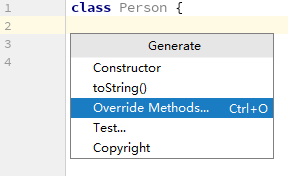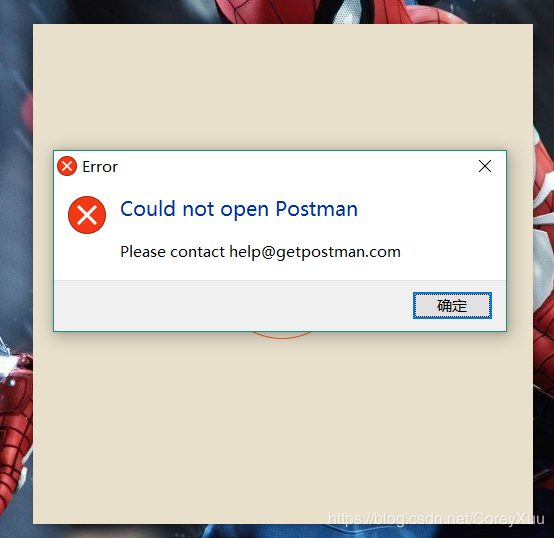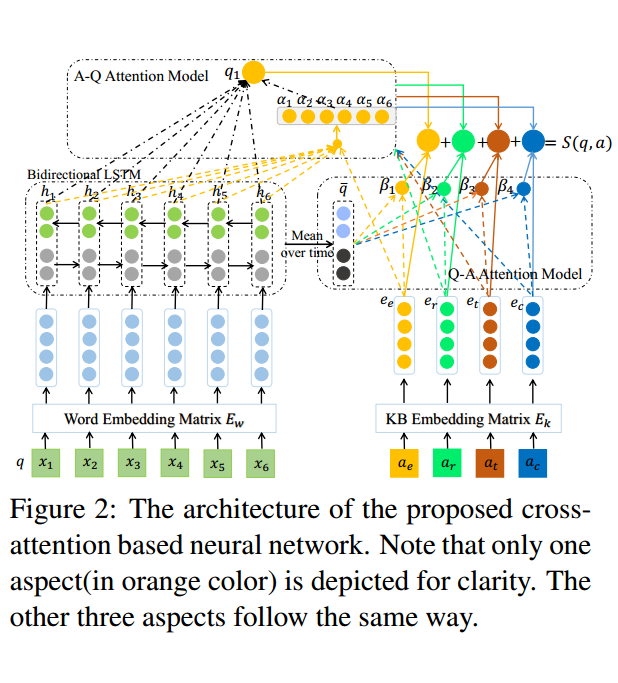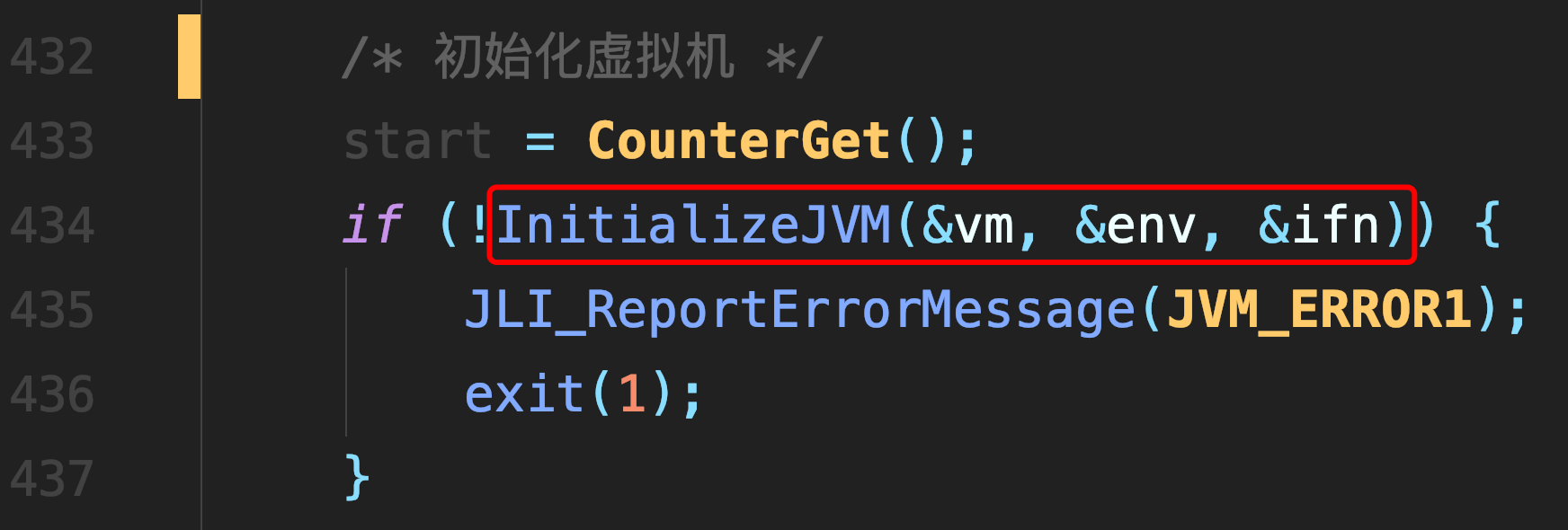# [转载] \$CF117B\$ 题解

``#include<bits/stdc++.h> using namespace std; inline int read() { int ret=0,f=1; char ch=getchar(); while(ch>'9'||ch<'0') { if(ch=='-') f=-1; ch=getchar(); } while(ch>='0'&&ch<='9') { ret=(ret<<1)+(ret<<3)+ch-'0'; ch=getchar(); } return ret*f; } int a,b,mod,ans; inline int solve(int a,int b,int mod) { int p=1000000000%mod; if(!p) return -1; if(b>=mod-1) return -1; int j=0; for(register int i=0;i<=min(a,mod);i++) { if(j>b) return i; j-=p; if(j<0) j+=mod; } return -1; } int main() { a=read(); b=read(); mod=read(); ans=solve(a,b,mod); if(ans==-1) printf("2\n"); else printf("1 %09d\n",ans); return 0; }``

##### 热门推荐
•浏览(655)
•浏览(565)
•浏览(548)
•浏览(488)
•浏览(433)
•浏览(424)
•浏览(381)
•浏览(367)
•浏览(362)
•浏览(357)